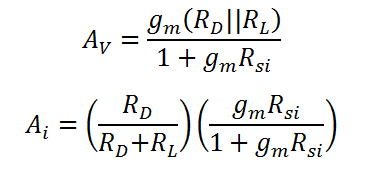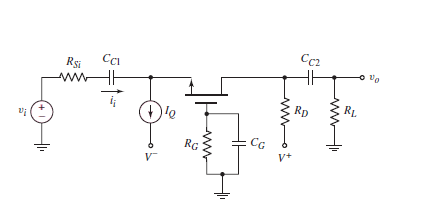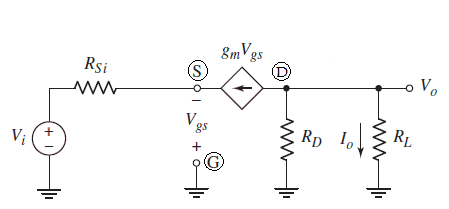# Small Signal Analysis of Common Gate (CG) MOSFET Amplifier

This CalcTown calculator calculates the small signal voltage gain of a Common Gate (CG) MOSFET amplifier, working in the saturation mode.

* Please note that body effect has been taken into consideration only for calculating the small signal output resistance and not for the current calculation in DC analysis.

K-Ohm
K-Ohm
K-Ohm
uA/V2

#### Result

uA/V
V/V
A/A